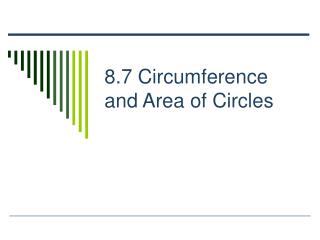DownloadDownload Presentation8.7 Circumference and Area of Circles

# 8.7 Circumference and Area of Circles

Download Presentation## 8.7 Circumference and Area of Circles

- - - - - - - - - - - - - - - - - - - - - - - - - - - E N D - - - - - - - - - - - - - - - - - - - - - - - - - - -
##### Presentation Transcript

1. 8.7 Circumference and Area of Circles

2. Definition • A circle is the set of all points in a plane that are the same distance from a given point, called the center of the circle. • A circle with center is called “circle ,” or .

3. Terms • The distance from the center to a point on the circle is the radius. • The distance across the circle, through the center, is the diameter. • The circumference of a circle is the distance around the circle.

4. r d Terms • radius • diameter • circumference c

5. Terms • For any circle, the ratio of the circumference to its diameter is denoted by the Greek letter π, or pi. • The number π is 3.14159…, which is an irrational number, which neither terminates nor repeats. • An approximation of 3.14 is used for π.

6. Circumference of a Circle • Words • Circumference = π (diameter) = 2π (radius) • Symbols • C = πd or C = 2πr

7. 4 in. Finding Circumference • Find the circumference of the circle. C = πd or C = 2πr C = 2πr = 2π(4) = 8π = 8(3.14) = 25.12 in.

8. Area of a Circle • Words • Area = π (radius)2 • Symbols • A = πr2

9. 7 cm Finding Area of a Circle • Find the Area of the Circle A = πr2 A = π(7)2 A = π(49) A = (3.14)(49) A = 153.94 cm2

10. r Using the Area of a Circle Find the radius of a circle with an area of 380 square feet. A = πr2 380 = πr2 380/ π = r² 120.96 ≈ r² 11 ft ≈ r

11. ө Central Angles An angle whose vertex is the center of a circle is a central angle of the circle. A region of a circle determined by two radii and a part of the circle is called a sector of the circle. r

12. Measurecentral angle Areasector = Areacircle Measurecircle Central Angles Because a sector is a portion of a circle, the following proportion can be used to find the area of a sector. r ө